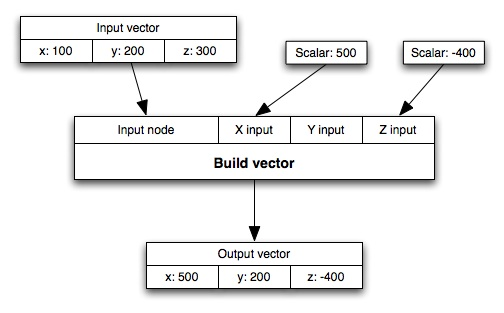# Build Vector

Node Description & Purpose:
The Build family of nodes allow you to construct colours or vectors. They let you input scalar values for each component. For the Build colour node you can input Red, Green and Blue values. For the Build vector node you can input X, Y and Z values.

The value from the Input node is used as the basis for building the new value. If you do not attach a node to one of the other inputs (Red/Green/Blue or X/Y/Z) then the value for that component is taken from the corresponding component of the Input node value. Here is an example, using a vector:

Input.x is 100
Input.y is 200
Input.z is 300

X scalar input is 500
Z scalar input is -400

The output vector will look like this:

Output.x is 500
Output.y is 200
Output.z is -400

As there is no scalar input for the Y component the y value from the input vector is used. Here is a diagram of the example above:Node Type: Function

Settings:

• The Build colour node has inputs which let you set the Red, Green and Blue values for the output colour.
• The Build vector node has inputs which let you set the X, Y and Z values for the output colour.

A scalar is a single number. 1, 200.45, -45, -0.2 are all examples of scalar values.

A vector is a set of three scalars, normally representing X, Y and Z coordinates. It also commonly represents rotation, where the values are pitch, heading and bank.